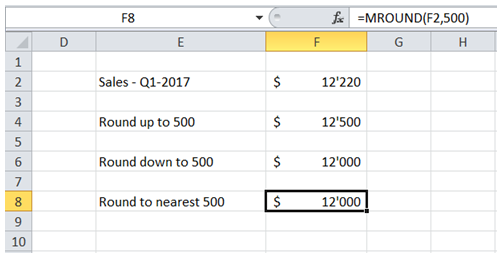## Round figures to nearest 500 in Excel

For example, for ease of review, I am interested in rounding the \$ values to nearest 500.

To do it in Excel, here is the answer:

a) Enter the formula =MROUND(F2,500) where F2 corresponds to sales figure.b) There are 2 other options -

i) For rounding down, enter the formula =FLOOR(F2,500) where F2 corresponds to sales figure.

ii) For rounding up, enter the formula =CEILING(F2,500) where F2 corresponds to sales figure.